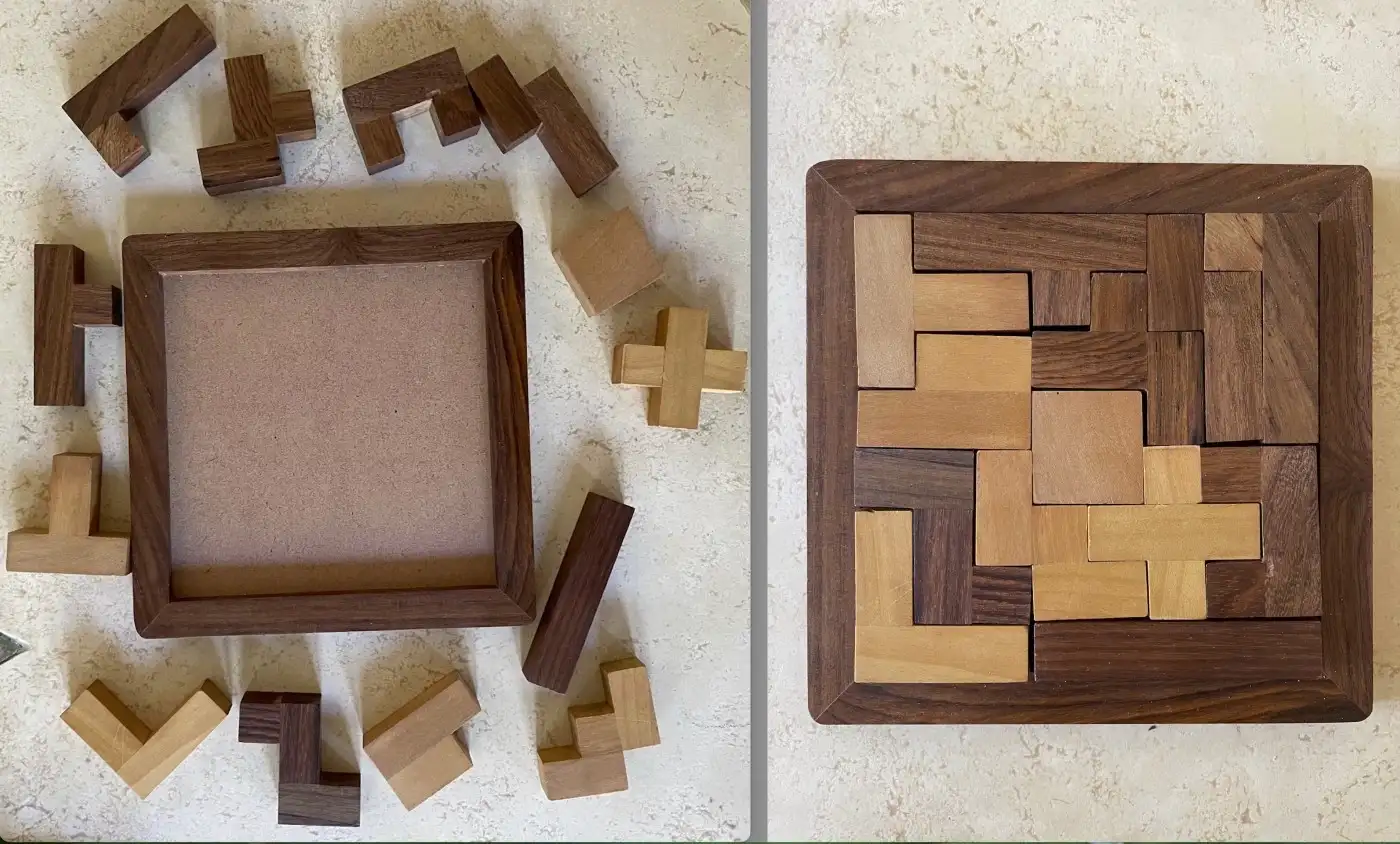• # 7 Missions of Calculator Series, Solving Puzles Fast and Tranding ChatGPTHello, checkiomates🐱‍👤!

At today's news CheckiO team presents a 🧮Calculator series of 7😮 missions to you! It follows with a usual portion of interesting reading! This time its about puzzle solving algorithm, speed performance comparing and ChatGPT for Python developers. So let's go!

In this series of missions you are going to build an elementary calculator. As an input, you get a sequence (string) of keys pressed , and, as the result of that function (string), you should show what will be shown on the screen when the last key is pressed.
🏁 MISSIONS:

Calculator-I by freeman_lex - In the first mission only digits and single signs (only +, -, =) between them are used.

```calculator("000000") == "0"
calculator("0000123") == "123"
calculator("12") == "12"```

Calculator-II by freeman_lex - In this mission the combinations of signs are presented.

```calculator("3+=") == "6"
calculator("3+2==") == "7"
calculator("3+-2=") == "1"```

Calculator-III by freeman_lex - Max digit space of 5 is added. It means that the maximum number you can enter is "99999".

```calculator("90000+10000=") == "error"
calculator("90000+10000-10000=") == "error"
calculator("90000+10000-10000") == "10000"```

Calculator-IV by freeman_lex - In the forth mission float numbers are allowed. The "." takes space as one digit, so max digit space is 4 for float number results. It means that the maximum float number you can enter is "9999.".

```calculator("0001.1000") == "1.100"
calculator("0001.1000-") == "1.1"
calculator("999.9999999+=") == "2000."```

Calculator-V by freeman_lex - In the fifth mission your function should work properly with additional operations: , /, // (integer division), % (modulo) and * (power) and their combinations with "=".

```calculator("10/2*2=") == "10."
calculator("10/=*=-=") == "0."
calculator("100//33**3=") == "27"```

Calculator-VI by freeman_lex - In the sixth mission are added such buttons as capital "B" (backspace) - delete previous character and capital "C" (clean) - delete all output, including error.

```calculator("123BB") == "1"
calculator("123C12*=B") == "14"
calculator("123BBBBBB") == "0"```

Calculator-VII by freeman_lex - In the seventh mission the memory should be implemented with such operations: capital "A" (add, +), capital "S" (subtract, -), capital "G" (get value) and capital "E" (erase memory).

```calculator("100A90S") == "0"
calculator("100A90SG") == "10"
calculator("100A90EG") == "0"```

Close look to the articles I mentioned!

💡ARTICLES:

Automating Puzzle Solving With Python - There are a lot of different puzzles at CheckiO and wil be more (maybe even with your help)! In this article the author explains, how he make a Python code, that solves pentamino puzzle. It's not only interesting to read, but may give you ideas and instruments to solve Checkio puzzles.Flood Fill: Recursion versus Iterative Solution - Using a flood fill game as an example, the author compares a speed performance of two type of solutions on different sixe of fields.

Top 10 Uses of ChatGPT for Python Programmers - ChatGPT becomes very popular recently. I am pretty sure, you have already heard about it. They say, some day it or it's descendant replace programmers! But for now, you may use it in your studies and work in following ways.

A bit of humour at the end!